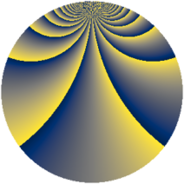# Properties

 Label 1140.2.cqLevel $1140$ Weight $2$ Character orbit 1140.cq Rep. character $\chi_{1140}(17,\cdot)$ Character field $\Q(\zeta_{36})$ Dimension $480$ Sturm bound $480$

# Related objects

## Defining parameters

 Level: $$N$$ $$=$$ $$1140 = 2^{2} \cdot 3 \cdot 5 \cdot 19$$ Weight: $$k$$ $$=$$ $$2$$ Character orbit: $$[\chi]$$ $$=$$ 1140.cq (of order $$36$$ and degree $$12$$) Character conductor: $$\operatorname{cond}(\chi)$$ $$=$$ $$285$$ Character field: $$\Q(\zeta_{36})$$ Sturm bound: $$480$$

## Dimensions

The following table gives the dimensions of various subspaces of $$M_{2}(1140, [\chi])$$.

Total New Old
Modular forms 3024 480 2544
Cusp forms 2736 480 2256
Eisenstein series 288 0 288

## Trace form

 $$480q + O(q^{10})$$ $$480q + 18q^{15} - 24q^{25} + 72q^{33} - 12q^{43} + 18q^{45} + 96q^{51} + 24q^{55} + 18q^{57} + 24q^{61} - 36q^{63} + 48q^{67} + 24q^{85} + 48q^{87} - 72q^{91} + 60q^{93} - 24q^{97} + O(q^{100})$$

## Decomposition of $$S_{2}^{\mathrm{new}}(1140, [\chi])$$ into newform subspaces

The newforms in this space have not yet been added to the LMFDB.

## Decomposition of $$S_{2}^{\mathrm{old}}(1140, [\chi])$$ into lower level spaces

$$S_{2}^{\mathrm{old}}(1140, [\chi]) \cong$$ $$S_{2}^{\mathrm{new}}(285, [\chi])$$$$^{\oplus 3}$$$$\oplus$$$$S_{2}^{\mathrm{new}}(570, [\chi])$$$$^{\oplus 2}$$